Aharoni, Ron

Strong matchings and covers ★★★

Author(s): Aharoni

Letbe a hypergraph. A strongly maximal matching is a matching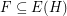so thatfor every matching. A strongly minimal cover is a (vertex) coverso thatfor every cover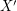.

Conjecture   Ifis a (possibly infinite) hypergraph in which all edges have sizefor some integer, thenhas a strongly maximal matching and a strongly minimal cover.

Keywords: cover; infinite graph; matching

Aharoni-Berger conjecture ★★★

Author(s): Aharoni; Berger

Conjecture   If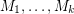are matroids on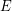andfor every partition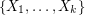of, then there existswithwhich is independent in every.

Keywords: independent set; matroid; partition

Strong colorability ★★★

Author(s): Aharoni; Alon; Haxell

Letbe a positive integer. We say that a graphis strongly-colorable if for every partition of the vertices to sets of size at mostthere is a proper-coloring ofin which the vertices in each set of the partition have distinct colors.

Conjecture   Ifis the maximal degree of a graph, thenis strongly-colorable.

Keywords: strong coloring